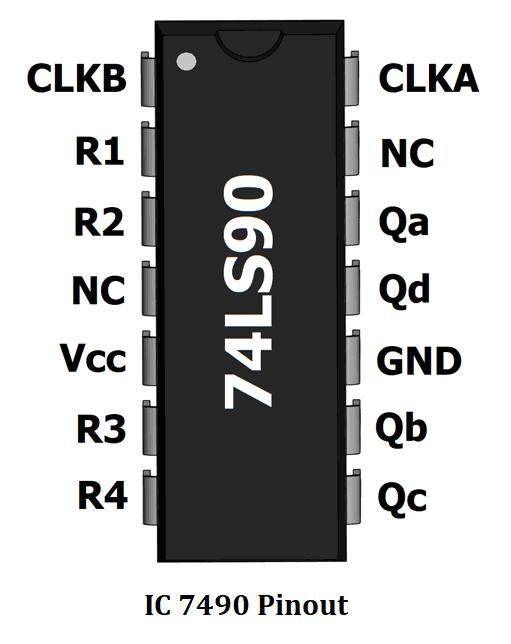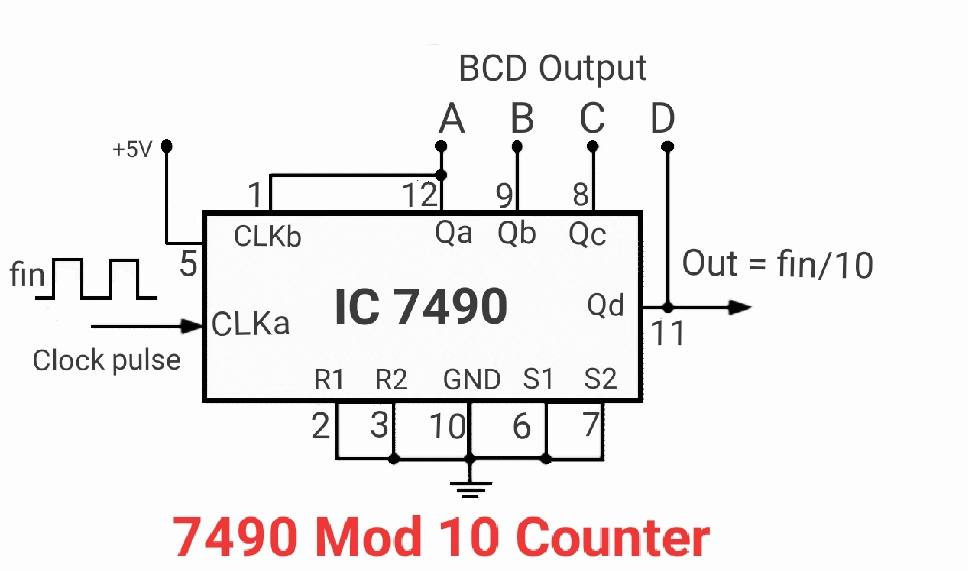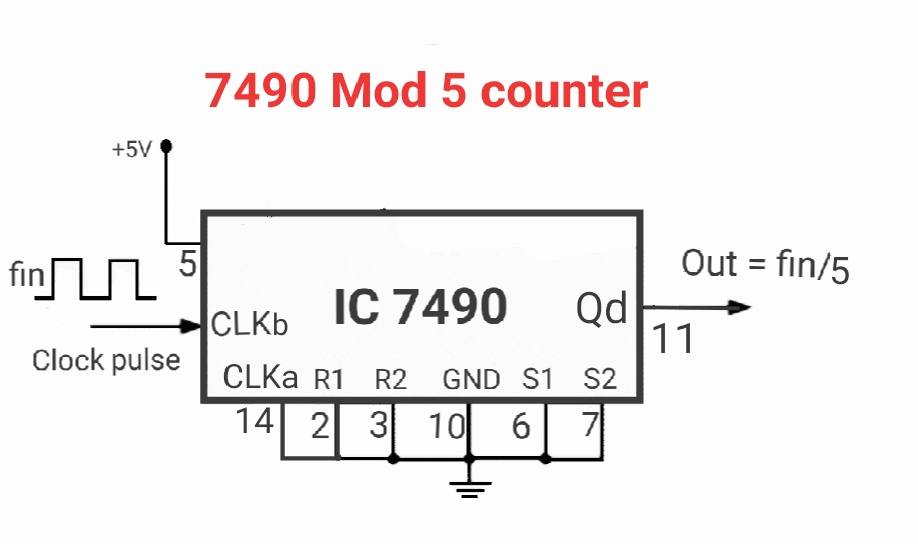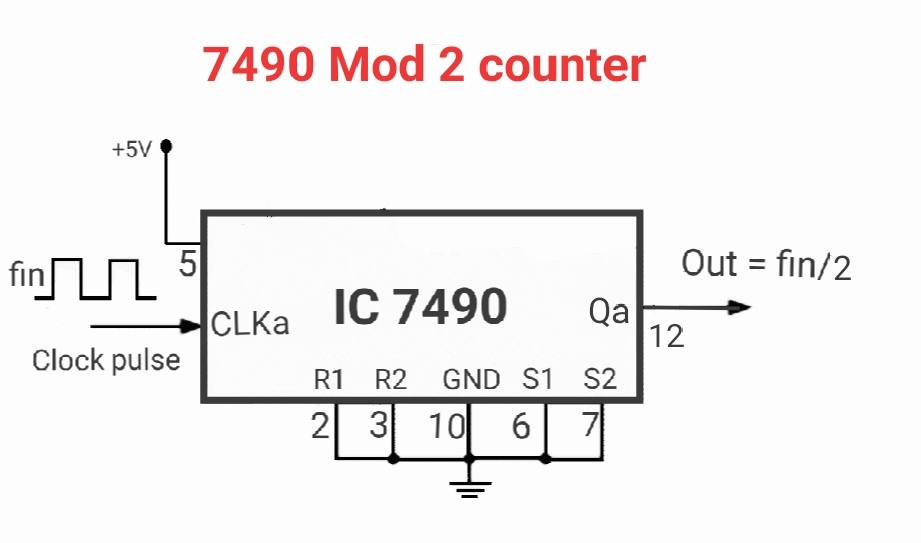# 7490 Decade Counter Circuit (Mod-10) Designing

IC 7490 is Asynchronous mod-10 Counter IC. In this article, we are going to study IC 7490 Decade Counter Circuit. IC 7490 is also known as BCD Counter, Decade Counter, and mod-10. These names are given based on the Functionality and Working Principle of IC 7490.

## Counter Designing using 7490 IC:

In the discussion of Counter ICs, some basic terms will be used a lot. That’s why we need to know some basic terminology while Designing any of Counter.

### Asynchronous Counters :

In Counters ICs there are so many Clock Inputs that are present based on the Internal Structure of Flip-Flops. If all the Clock Input of the Flip-Flops are not driven(controlled) by the same Clock Signals(Pulse) then the Counter is Defined as Asynchronous Counters. ICs 7490, ICs 7493, etc. are examples of Asynchronous Counters.

### Synchronous Counters :

If all the Clock Input of the Flip-Flops are driven(controlled) by the same Clock Signals(Pulse) then the Counter is Defined as Synchronous Counters.  ICs 74160, ICs 74163, ICs 74168, ICs 74169 etc. are Synchronous Counters.

#### Clock Signals(Pulse):

It is a continuous high-low signal and is represented as a square waveform.

#### BCD :

BCD Stands for Binary Coded Decimal. It Simply means the Decimal number is replaced by their Equivalent Binary Code. for e.g. the Decimal number 10 is replaced by there equivalent Binary Code 1010

#### mod-n :

mod represent Modulus of Counter and n belongs to an integer. Modulus of Counter is the total number of Unique States it should pass through in one complete Counting Cycle. It means if we need to design mod-6 counter then it should pass through six Unique States in one Complete Counting Cycle. That is 0000(0),  0001(1),  0010(2),  0011(3),  0100(4),  0101(5), then again come back to 0000(0).

#### Frequency Division :

As we know that each and every Counter Circuit is made up of Flip-Flops. if common Clock Signals do not trigger the Clock Inputs of all Flip-Flops then the output produced by such Sequential Circuit is always reduced by 50% of its previous Cycle. If you observe the Following figure carefully your all doubts will get clear automatically related to Frequency Division. IC 7490 can be used as a frequency divider circuit. It can divide the input frequency by 2, 5, and 10.#### Sequential Circuit

If any Circuit starts its Counting in series i.e., the count may be in increasing order or decreasing order. ICs 7490 decade counter is an example of a Sequential Circuit. Because its Counting Sequence is 0,1,2,3,4,5,6,7,8,9. its Count always in the upward direction(in increasing order) that’s why it is also known as Up Counter.

Now we are going to a little deeper part and we will study its basic structure known as Block Diagram. Before going to that part I want to tell you one thing don’t confuse between Block Diagram and Pin Diagram(Pinouts) of any ICs. There is a huge difference between Block Diagram and Pin Diagram later on we will see that.

### Block Diagram of 7490 counter IC :

Let us understand the Block Diagram of ICs 7490 which is given in the following figure. And this is one of the major parts of this article so keep reading with high concentration.Now let us start a brief introduction of ICs 7490. Start with its input terminals

#### VCCand GND :

VCC and GND are the input pins of ICs 7490 which is used to give power supply to the ICs. These input terminals generally present in all devices to give power supply to the devices.  VCC stands for Voltage Common Collectors and in most cases, it is referred to as high voltage with respect to GND(Ground) terminal. It may be positive and negative with respect to GND(Ground).

#### Clock Inputs (Aand B) :

The terminal represented as A and B in the above figure are knowns as Clock Inputs. These inputs terminal used to provide Clock Signals to the ICs. It presents in all Counter ICs for the same purpose.

#### Set Inputs (S1 and S2) :

The input terminals S1 and S2 are knowns as Set Input terminal because it is used to set or load any data(state) to the ICs. Here data(state) means any random number say 0011(3). If we want to start our counting from 0000(3) then we need to Connect it with VCC or Logic 1  because Set Input is Active High input Set Inputs Play its magical role and it forces IC to start its Counting from 0000(3). By default, ICs 7490 Start its Counting from 0000(0) and pre-set input is absent in this IC so we can’t use this Set Input(S1 and S2). That’s why we always connect it with GND(Ground).

### IC 7490 Decade Counter Circuit Pin Diagram:

IC 7490 is a 14 pin DIP(dual inline package) ic. The pin description of 7490 is as follows,### Working of 7490 Decade Counter Circuit:Its a BCD counter it can count from 0 to 9 (10 states), hence it is called a mod-10 counter. It has two separate counters, a mod 2 counter, and another mod 5 counter. Together they form a mod 10 counter. The first bit is given by mod 2 counter its output is used as a clock for the second mod-5 counter. Give clock to clkB to use as mod 5 counter and give clock to clkA to use as mod 2 counter.

#### 7490 Mod counter :When the clock is given to the mod 2 part of the ic it counts 0 and 1 it’s output Qa is given to the mod 5 part of the ic. By combining both the counters it counts from 0 to 9 in BCD format.

Other than this there are two reset pins R0, R1, and two set pins S0, S1. Making the reset pin high or set pin low counter will be reset to 0000 (0). If we make reset pin low and set pin high the counter will be set to 1001(9), the highest count.We can increase the count to 99 (mod-100) or 999 (mod-1000) as per our requirements. The highest output of the previous IC is used as a clock to the next ic. It can work as a mod-10 counter by connecting the 2nd clock input(pin 1) and QA. And connecting all rest pins to the ground and giving pulse to pin 1. It can work as a mod-6 counter by supplying pulse at input 1 and grounding reset pins R3 and R4 and connecting QA with input 2.

7490 IC can work as BCD (binary coded decimal) counter. It is used to store decimal digits in the form of 4-bit binary numbers.

This site uses Akismet to reduce spam. Learn how your comment data is processed.